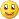# Alpha Beta Price Channel Indicator or System250

Hi all,

Any coders or traders here used ALPHA BETA PRICE CHANNEL indicator or have any mq4 codes of it. Any help or suggestions appreciated250

Alpha Beta Price Channel - Details

Alpha indicator quantifies the residual risk that an investor takes as a result of investing in a fund rather than in a market index. This indicator represents the difference between a mutual fund's actual performance and the performance that would be expected based on the level of risk taken by the fund's manager.

If a fund produced the expected return for the level of risk assumed, the fund is said to have an Alpha of zero. A positive Alpha indicates a return greater than expected for the risk taken. A negative Alpha indicates the manager has not adequately rewarded investors for the risks taken.

Alpha = [ (sum of y) - {b * (sum of x)} / n ]:

n - number of observations

b - Beta of the fund

x - rate of return for the benchmark index (often, but not always, the S&P 500)

y - rate of return for the fund

In general, the Alpha-Beta Trend Channel study uses the standard deviation of price variation to establish two trend lines, one above and one below the moving average of a price field. This creates a channel (band) where the great majority of price field values will occur.

The Beta Coefficient is the measure of the systematic risk of a security. This indicator is used to illustrate the relative volatility of a security (or portfolio) in comparison with the market. As the benchmark of this measurement, the market is defined of having a beta of 1.0.

Beta value higher than 1.0 indicates of a security that is more volatile than the market. A Beta less than 1.0 indicates less volatile than the market. If the Beta = 1.0, the security's price is said to move along with the market.

Beta2

There is the Beta2 coefficient. The difference between Beta and Beta2 being that instead of using the Simple Rate of Change, Beta2 uses the Moving Average of the Rate of Change in its calculation.

With either form of Beta, securities with a value higher than 1.0 will be more volitile than the market. A Beta less than 1.0 is said to be less volatile than the market.

In general, the Alpha-Beta Trend Channel study uses the standard deviation of price variation to establish two trend lines, one above and one below the moving average of a price field. This creates a channel (band) where the great majority of price field values will occur.250

Done it myself

Hi all,

I have done this indicator myself though need little code fixing i hope i can do it myself and thanks for all who spend there precious time to click this thread302

Hi

Well

I would like to have a glance on the code for improving it

email jaguar1637 at yahoo dot com

regards